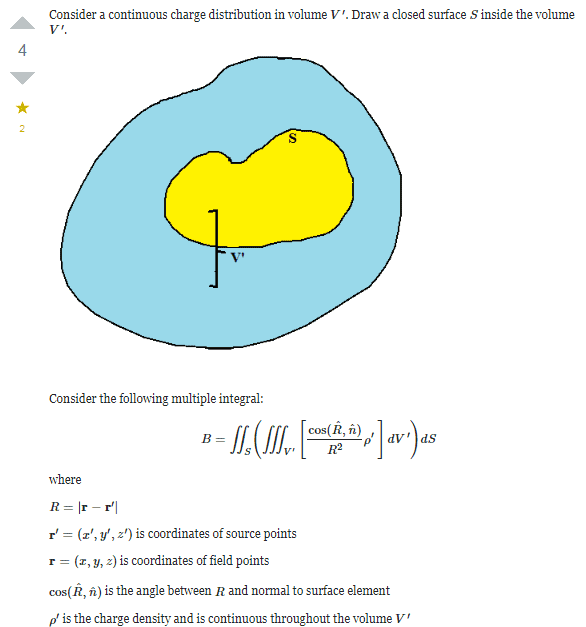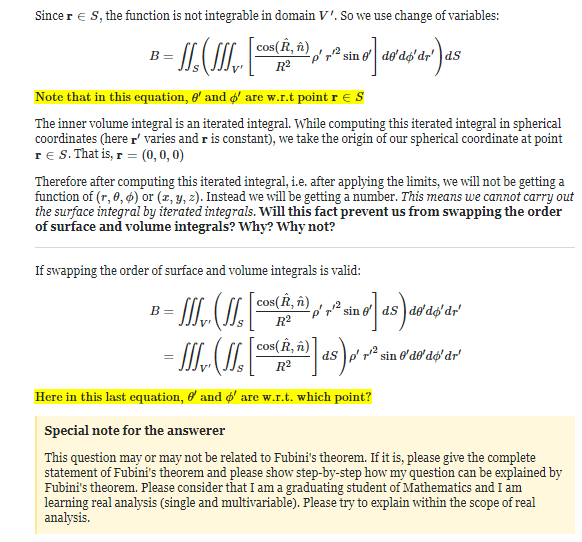# Swapping the order of surface and volume integrals

• I

TL;DR Summary
The integral is not iterated integral. Will this fact prevent us from swapping the order of surface and volume integrals? Why? Why not?•Delta2

Which fact? What do you mean by "not iterated integral"?

There is nothing which wouldn't allow us to swap integrals in a finite case over a smooth function. One has only to be aware of the fact, that integral limits might change!

This is a continuation of his previous thread I think:

The consensus there was: While you can swap the order of integration of individual integrals with appropriate change of limits, you can't simply extend that to swap a surface integral with a volume integral.

https://mathinsight.org/double_integral_change_order_integration_examples
From Fubini's theorem:

https://en.wikipedia.org/wiki/Fubini's_theorem
Failure of Fubini's theorem for non-integrable functions
Fubini's theorem tells us that (for measurable functions on a product of σ-finite measure spaces) if the integral of the absolute value is finite, then the order of integration does not matter; if we integrate first with respect to x and then with respect to y, we get the same result as if we integrate first with respect to y and then with respect to x. The assumption that the integral of the absolute value is finite is "Lebesgue integrability", and without it the two repeated integrals can have different values.

A simple example to show that the repeated integrals can be different in general is to take the two measure spaces to be the positive integers, and to take the function f(x,y) to be 1 if x=y, −1 if x=y+1, and 0 otherwise. Then the two repeated integrals have different values 0 and 1.

Another example is as follows for the functionThe iterated integralsandhave different values. The corresponding double integral does not converge absolutely (in other words the integral of the absolute value is not finite):I think if you rewrite your equations using the explicit integrals you will begin to see that you can't do what you want.

I not knowledgeable enough to help you with that. @fresh_42 @Mark44 or @HallsofIvy might be able to explain it better.

I guess ##\frac{1}{R^2}## is the problem here. If we integrate the surface (##R>0## fixed), we get a certain value. This has no poles anymore, so integrating the volume makes no problems. If we first integrated volume, we had to run over the singularity at ##R=0## and everything after that is meaningless. So the integrand isn't "nice" enough in this case. Fubini requires finite, i.e. measurable integrals.

What do you mean by "not iterated integral"?
One like this: ##\int_V f(x, y, z)dV##
This can also be written as ##\iiint_V f(x, y, z)dV##. I've seen both styles in textbooks.

Here is an example of an iterated integral:
##\int_{y=0}^\pi\int_{x = 0}^\pi \sin(x + y)dx~dy##

One like this: ##\int_V f(x, y, z)dV##
This can also be written as ##\iiint_V f(x, y, z)dV##. I've seen both styles in textbooks.

Here is an example of an iterated integral:
##\int_{y=0}^\pi\int_{x = 0}^\pi \sin(x + y)dx~dy##
I don't see a difference. What is a "not iterated integral"? Is it simple versus multiple? ##\int_V f\,dV## and ##\int_{V_z}\int_{V_y}\int_{V_x} f\, dx\, dy\, dz ## is the same thing.

What is a "not iterated integral"?
One that is not an iterated integral...Is it simple versus multiple? ##\int_V f\,dV## and ##\int_{V_z}\int_{V_y}\int_{V_x} f\, dx\, dy\, dz ## is the same thing.
Maybe, or maybe not. A double integral such as ##\int_R f\,dA## (where R is the region in the plane over which integration is to be performed) could be rewritten as two different iterated integrals: one in Cartesian form or one in polar form.

I would call this multiple or nested. It's not really an iteration. So the answer to my question is: A not iterated integral is a single integral. That means ##\int dV## versus ##\int dx\,dy\,dz## is more a linguistic issue than a mathematical.

I would call this multiple or nested. It's not really an iteration. So the answer to my question is: A not iterated integral is a single integral. That means ##\int dV## versus ##\int dx\,dy\,dz## is more a linguistic issue than a mathematical.
I disagree. No calculus textbook that I've ever seen would write an integral like this: ##\int dx\,dy\,dz##.

Again, it's the difference between this triple integral ##\int_D f(x, y, z) dV## or ##\iiint_D f(x, y, z) dV## (not iterated) and this iterated integral ##\int_{z = z_1}^{z_2}\int_{y = y_1}^{y_2}\int_{x = x_1}^{x_2} f(x, y, z) dx~dy~dz##.

I disagree. No calculus textbook that I've ever seen would write an integral like this: ##\int dx\,dy\,dz##.
Yes, I forgot to triple the integration symbol.
Again, it's the difference between this triple integral ##\int_D f(x, y, z) dV## or ##\iiint_D f(x, y, z) dV## (not iterated) and this iterated integral ##\int_{z = z_1}^{z_2}\int_{y = y_1}^{y_2}\int_{x = x_1}^{x_2} f(x, y, z) dx~dy~dz##.
Yes, but how is this not purely notational? To distinguish it linguistically appears hair splitting to me.

Yes, but how is this not purely notational?
Because the iterated form contains information about the order in which integration is to be performed, in addition to possibly distinguishing between Cartesian coordinates or polar/cylindrical coordinates are to be used.

••